## Polygamma Function

The polygamma function is sometimes denoted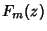, and sometimes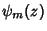. Innotation,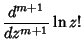(1)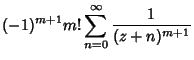(2)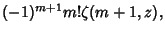(3)

where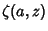is the Hurwitz Zeta Function. In the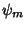Notation (the form returned by the PolyGamma[m,z] function in Mathematica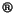; Wolfram Research, Champaign, IL),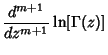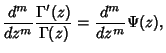(4)

where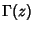is the Gamma Function and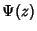is the Digamma Function.is therefore related toby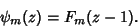(5)

The function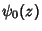is equivalent to the Digamma Function. Note that Morse and Feshbach (1953) adopt a notation no longer in standard use in which Morse and Feshbach'sis equal to the above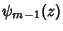.

The polygamma function obeys the Recurrence Relation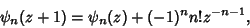(6)

the reflection Formula(7)

and the multiplication Formula,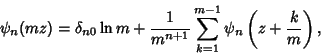(8)

where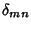is the Kronecker Delta.

In general, special values for integral indices are given by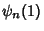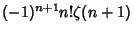(9)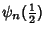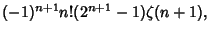(10)

giving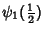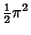(11)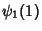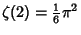(12)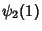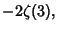(13)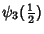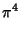(14)

and so on.

R. Manzoni has shown that the polygamma function can be expressed in terms of Clausen Functions for Rational arguments and integer index. Special cases are given by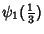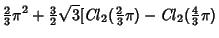(15)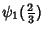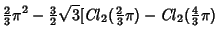(16)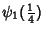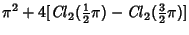(17)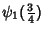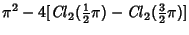(18)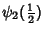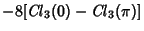(19)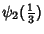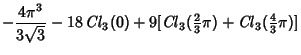(20)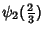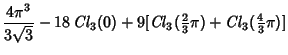(21)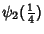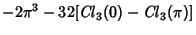(22)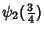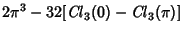(23)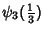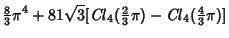(24)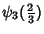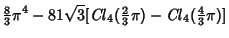(25)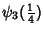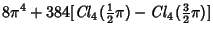(26)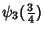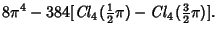(27)

References

Abramowitz, M. and Stegun, C. A. (Eds.). Polygamma Functions.'' §6.4 in Handbook of Mathematical Functions with Formulas, Graphs, and Mathematical Tables, 9th printing. New York: Dover, p. 260, 1972.

Adamchik, V. S. Polygamma Functions of Negative Order.'' Submitted to J. Symb. Comput.

Arfken, G. Digamma and Polygamma Functions.'' §10.2 in Mathematical Methods for Physicists, 3rd ed. Orlando, FL: Academic Press, pp. 549-555, 1985.

Davis, H. T. Tables of the Higher Mathematical Functions. Bloomington, IN: Principia Press, 1933.

Kolbig, V. The Polygamma Function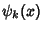for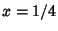and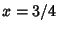.'' J. Comp. Appl. Math. 75, 43-46, 1996.

Morse, P. M. and Feshbach, H. Methods of Theoretical Physics, Part I. New York: McGraw-Hill, pp. 422-424, 1953.